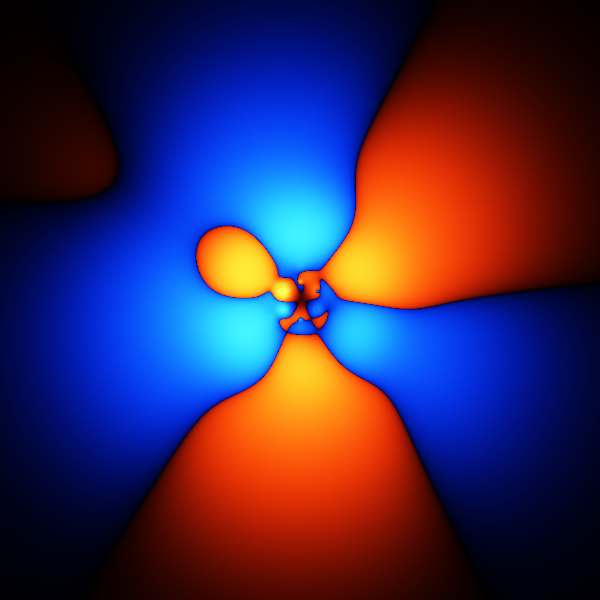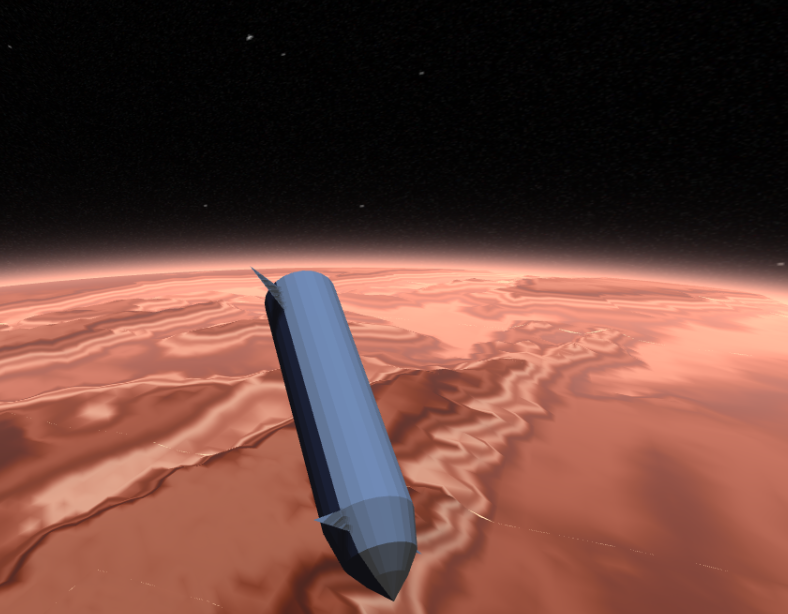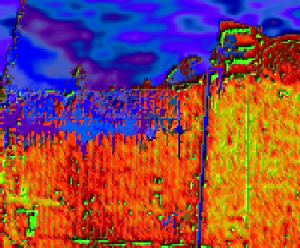﻿ Maths Exam @Template

### Maths Exam

1. Simplify 1

 Simplify the following expression: $$\left(2 - 6\right)\left(-1 - -6\right) - {\left(3 - 2\right)}^{2}$$ Correct Answer $$-21$$ or -21.0000

2. Simplify 2

 Simplify the following expression: $${\left(2(-6)5\right)}^{\left(\frac{-6}{5} - 1\times1\right)}$$ Correct Answer $${(-60)}^{\frac{-11}{5}}$$ or NaN

3. Fractions 1

 Simplify the following expression: $$\frac{5}{2} - \frac{2}{5}$$ Correct Answer $$\frac{21}{10}$$ or 2.1

4. Fractions 2

 Simplify the following expression: $$\frac{\frac{2}{6}}{\frac{1}{3}}$$ Correct Answer $$1$$ or 1.0

5. Significant figures

 1 Write the following to 5 significant figures: 2.8397859Correct Answer 2.8398 or $$2.8398\times10^{0}$$ 2 Write the following to 4 significant figures: 848.40737Correct Answer 848.4 or $$8.484\times10^{2}$$

6. Standard Form

 1 Write the following in standard form to 4 significant digits: 0.000210263Correct Answer $$2.103\times10^{-4}$$ 2 Write the following in standard form to 4 significant digits: 0.284492Correct Answer $$2.845\times10^{-1}$$

7. Significant figures

 1 Write the following in preferred standard form to 4 significant digits: 0.000574249Correct Answer $$574.2\times10^{-6}$$ 2 Write the following in preferred standard form to 3 significant digits: 0.1748Correct Answer $$175\times10^{-3}$$

8. Simplify 2

 Simplify the following expression: $$\frac{2\times4}{-4(-5)}2(-6)1$$ Correct Answer $$\frac{-24}{5}$$ or -4.80000

9. Simplify 1

 Simplify the following expression: $$2\times2\times4\left(-5(-1) + -1 - 5\right)$$ Correct Answer $$-16$$ or -16.0000

10. Simplify 2

 Simplify the following expression: $${\left(2 + 5\right)}^{2} - \frac{4 - -4}{-6 + -5}$$ Correct Answer $$\frac{547}{11}$$ or 49.7273

## Other Articles:## Benzene QMC Gallery

A gallery of images from an attempt to model the benzene ground state using a variational and diffusion monte carlo method.## Mars Reentry

A ThreeJS simulation of mars reentry in a spaceship.## Simulation of flooding in new orleans

A not-very-accurate simulation of the flooding in new orleans.